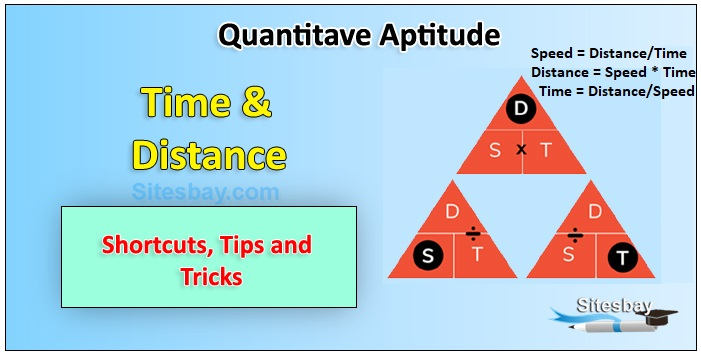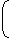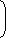# Time and Distance Problem

## Time and Distance Problem

Speed: Speed is defined as the distance covered by an object in unit time.## Formula

 speed = distance cover time taken

### Some Important Facts

• Distance travelled is proportional to the speed of the object if the time is kept constant.
• Distance travelled is proportional to the time taken if speed of object is kept constant.
• Speed is inversely proportional to the time taken if the distance covered is kept constant.
• If the ratio of two speeds for same distance is a:b then the ratio of time taken to cover the distance is b:a

## Relative Speed

If two objects are moving in same direction with speeds of x and y then their relative speed is

## Formula

(x - y)

If two objects are moving is opposite direction with speeds of x and y then their relative speed is

(x + y)

## km/hr to m/sec conversion

 x km/hr =x x 5m/sec. 18

## m/sec to km/hr conversion

 x m/sec =x x 18km/hr. 5

### Important Shortcut Formulas

If some distance is travelled at x km/hr and the same distance is travelled at y km/hr then the average speed during the whole journey is given by

## average speed

 average speed =2xykm/hr x+y

If a person travels a certain distance at x km/hr and returns at y km/hr, if the time taken to the whole journey is T hours then the one way distance is given by

## one way distance

 one way distance = txykm x+y

If two persons A and B start their journey at the same time from two points P and Q towards each other and after crossing each other they take a and b hours in reaching Q and P respectively, then

### Time and Distance Shortcut Methods

Rule 4: If the same distance is covered at two different speeds S1 and S2 and the time taken to cover the distance are T1 and T2, then the distance is given by